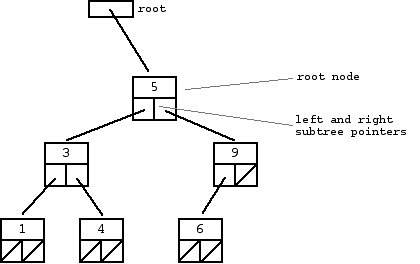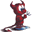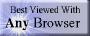music# trees

## summary

This chapter looks at trees.

## free computer programming text book project

If you like the idea of this project,# trees

This chapter looks at trees.

### basic terminology

A tree is any connected undirected graph with no simple circuits.

A rooted tree is a data structure that consists of nodes (data elements or vertices) with zero, one, or multiple child pointers or references (directed edges) to other elements. Any given node (other than the root) can have only (exactly) one other node pointing to or referencing it.The root is the node (vertex) at the “top” of a rooted tree. In the example, the root node is A.

A parent is a node that points to one or more other nodes. That is, a parent is a vertex that has a unique directed edge to another vertex. In the example, A is the parent of B, C, and D. Every node except the root node (A) has a parent.

A child is a node that is pointed to or referenced by another node. In the example, B, C, and D are child nodes of A.

Siblings are nodes (vertices) that share the same parent. In the example, H, I, and J are siblings (sharing the common parent D)

A descendant is a node that can be reached by following a path of child nodes from a particular node. In the example, E and F are both child nodes and descendants of B. G and K are both descendants of C, but G is a child of C while K is a child of G.

An ancestor is any node that is higher up in the tree and has a path through a child or series of children nodes to a particular node. In the example, A, D, and I are the ancestor nodes of M.

A leaf is a node (vertex) that does not have any children. The leaves of a tree are all nodes that do not have any children. In the example, the leaves are E, F, H, J, K, L, and M.

An internal vertex is a node (vertex) that has at least one child. In the example, the internal vertices are B, C, D, G, and I.

A binary tree is a special kind of tree where each node has no more than two children (that is, every node has only zero, one, or two children).

A binary search tree (or BST) is a special kind of binary tree where the stored value of any node’s left child is always less than or equal to the node’s own stored value and the stored value of any node’s right child is always greater than or equal to the node’s own stored value.

When you hear a general reference to the word “tree”, chances are that the person is usually talking about a binary tree and often means a binary search tree.

A binary search tree is one of the fastest data structures for searching. Lookup time is O(log2(n)).

A balanced binary search tree (or balanced BST) is a special kind of binary search tree where every non-leaf node has approximately the same number of descendant nodes on its right and left sides.

The time to delete or insert a node into a balanced BST is O(log2(n)).

### basic theorems

An undirected graph is a tree if and only if there is a unique simple path between any two of it vertices.

## Stanford introduction

This [the following section until marked as end of Stanford University items] is article #110 in the Stanford CS Education Library. This and other free CS materials are available at the library (http://cslibrary.stanford.edu/). That people seeking education should have the opportunity to find it. This article may be used, reproduced, excerpted, or sold so long as this paragraph is clearly reproduced. Copyright 2000-2001, Nick Parlante, nick.parlante@cs.stanford.edu. [Stanford items are marked by a very light Stanford cardinal background.]

## Section 1 -- Introduction To Binary Trees

A binary tree is made of nodes, where each node contains a “left” pointer, a “right” pointer, and a data element. The “root” pointer points to the topmost node in the tree. The left and right pointers recursively point to smaller “subtrees” on either side. A null pointer represents a binary tree with no elements -- the empty tree. The formal recursive definition is: a binary tree is either empty (represented by a null pointer), or is made of a single node, where the left and right pointers (recursive definition ahead) each point to a binary tree.A “binary search tree” (BST) or “ordered binary tree” is a type of binary tree where the nodes are arranged in order: for each node, all elements in its left subtree are less-or-equal to the node (<=), and all the elements in its right subtree are greater than the node (>). The tree shown above is a binary search tree -- the “root” node is a 5, and its left subtree nodes (1, 3, 4) are <= 5, and its right subtree nodes (6, 9) are > 5. Recursively, each of the subtrees must also obey the binary search tree constraint: in the (1, 3, 4) subtree, the 3 is the root, the 1 <= 3 and 4 > 3. Watch out for the exact wording in the problems -- a “binary search tree” is different from a “binary tree”.

The nodes at the bottom edge of the tree have empty subtrees and are called “leaf” nodes (1, 4, 6) while the others are “internal” nodes (3, 5, 9).

### Binary Search Tree Niche

Basically, binary search trees are fast at insert and lookup. The next section presents the code for these two algorithms. On average, a binary search tree algorithm can locate a node in an N node tree in order lg(N) time (log base 2). Therefore, binary search trees are good for “dictionary” problems where the code inserts and looks up information indexed by some key. The lg(N) behavior is the average case -- it’s possible for a particular tree to be much slower depending on its shape.

### Strategy

Some of the problems in this article use plain binary trees, and some use binary search trees. In any case, the problems concentrate on the combination of pointers and recursion. (See the articles linked above for pointer articles that do not emphasize recursion.)

For each problem, there are two things to understand…

• The node/pointer structure that makes up the tree and the code that manipulates it
• The algorithm, typically recursive, that iterates over the tree

When thinking about a binary tree problem, it’s often a good idea to draw a few little trees to think about the various cases.

### Typical Binary Tree Code in C/C++

As an introduction, we’ll look at the code for the two most basic binary search tree operations -- lookup() and insert(). The code here works for C or C++. … In Java, the key points in the recursion are exactly the same as in C or C++. In fact, I created the Java solutions by just copying the C solutions, and then making the syntactic changes. The recursion is the same, however the outer structure is slightly different.

In C or C++, the binary tree is built with a node type like this…

struct node {
int data;
struct node* left;
struct node* right;

}

{NOTE; the original Stanford document separated the C/C++ and the Java discusssions. The material is interwoven here because this book uses the approach of teaching abasic concept and then showing it in multiple languages.]

### Pointer Changing Code

There is a common problem with pointer intensive code: what if a function needs to change one of the pointer parameters passed to it? For example, the insert() function below [moved to tree insert] may want to change the root pointer. In C and C++, one solution uses pointers-to-pointers (aka “reference parameters”). That’s a fine technique, but here we will use the simpler technique that a function that wishes to change a pointer passed to it will return the new value of the pointer to the caller. The caller is responsible for using the new value. Suppose we have a change() function that may change the the root, then a call to change() will look like this…

// suppose the variable "root" points to the tree
root = change(root);

We take the value returned by change(), and use it as the new value for root. This construct is a little awkward, but it avoids using reference parameters which confuse some C and C++ programmers, and Java does not have reference parameters at all. This allows us to focus on the recursion instead of the pointer mechanics. (For lots of problems that use reference parameters, see CSLibrary #105, Linked List Problems, http://cslibrary.stanford.edu/105/).

### Binary Tree Problems

Here are 14 binary tree problems in increasing order of difficulty. Some of the problems operate on binary search trees (aka “ordered binary trees”) while others work on plain binary trees with no special ordering. The next section, Section 3, shows the solution code in C/C++. Section 4 gives the background and solution code in Java. The basic structure and recursion of the solution code is the same in both languages -- the differences are superficial.

Reading about a data structure is a fine introduction, but at some point the only way to learn is to actually try to solve some problems starting with a blank sheet of paper. To get the most out of these problems, you should at least attempt to solve them before looking at the solution. Even if your solution is not quite right, you will be building up the right skills. With any pointer-based code, it’s a good idea to make memory drawings of a a few simple cases to see how the algorithm should work.

[These problems are repeated in separate subchapters to allow additional commentary. In the original file the problems and the solutions were separated into differetn sections. Here they are grouped together. It is strongly recommended that readers follow Dr. Nick Parlante’s advice and attempt to solve the problems before reading the answers.]

This [the quoted passages above] is article #110 in the Stanford CS Education Library. This and other free CS materials are available at the library (http://cslibrary.stanford.edu/). That people seeking education should have the opportunity to find it. This article may be used, reproduced, excerpted, or sold so long as this paragraph is clearly reproduced. Copyright 2000-2001, Nick Parlante, nick.parlante@cs.stanford.edu. [Stanford items are marked by a very light Stanford cardinal background.]

### end of Stanford introduction

“36 Access types allow the construction of linked data structures. A value of an access type represents a reference to an object declared as aliased or to an object created by the evaluation of an allocator. Several variables of an access type may designate the same object, and components of one object may designate the same or other objects. Both the elements in such linked data structures and their relation to other elements can be altered during program execution. Access types also permit references to subprograms to be stored, passed as parameters, and ultimately dereferenced as part of an indirect call.” —:Ada-Europe’s Ada Reference Manual: Introduction: Language Summary See legal information

### other

“Rule 3. Fancy algorithms are slow when n is small, and n is usually small.ÊFancy algorithms have big constants. Until you know that n is frequently going to be big, don’t get fancy. (Even if n does get big, don’t tune for speed until you’ve measured, and even then don’t unless one part of the code overwhelms the rest..) For example, binary trees are always faster than splay trees for workaday problems.” —Rob Pike, Notes on Programming in C, February 21, 1989

“Pointers also require sensible notation. np is just as mnemonic as nodepointer if you consistently use a naming convention from which np means “node pointer” is easily derived.” —Rob Pike, Notes on Programming in C, February 21, 1989

# free music player coding example

Coding example: I am making heavily documented and explained open source code for a method to play music for free — almost any song, no subscription fees, no download costs, no advertisements, all completely legal. This is done by building a front-end to YouTube (which checks the copyright permissions for you).

View music player in action: www.musicinpublic.com/.

Create your own copy from the original source code/ (presented for learning programming).

## view text bookHTML file

Because I no longer have the computer and software to make PDFs, the book is available as an HTML file, which you can convert into a PDF.

 Tweets by @osdata

## free computer programming text book project

Building a free downloadable text book on computer programming for university, college, community college, and high school classes in computer programming.

If you like the idea of this project,

send donations to:
Milo
PO Box 1361
Tustin, California 92781

Supporting the entire project:

If you have a business or organization that can support the entire cost of this project, please contact Pr Ntr Kmt (my church)

Some or all of the material on this web page appears in theThis web site handcrafted on Macintoshcomputers using Tom Bender’s Tex-Edit Plusand served using FreeBSD.†UNIX used as a generic term unless specifically used as a trademark (such as in the phrase “UNIX certified”). UNIX is a registered trademark in the United States and other countries, licensed exclusively through X/Open Company Ltd.

Names and logos of various OSs are trademarks of their respective owners.